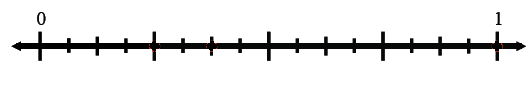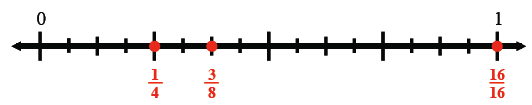### Home > ACC6 > Chapter 6 Unit 6 > Lesson CC1: 6.1.3 > Problem6-40

6-40.

Arrange each of these fractions on a number line: $\frac { 5 } { 8 } , \frac { 3 } { 16 } , \frac { 3 } { 8 } , \frac { 16 } { 16 } , \frac { 1 } { 4 } , \frac { 1 } { 8 } , \frac { 3 } { 4 } , \frac { 7 } { 8 }$.

Arrange the fractions on the number line below. It is divided into sixteenths.
This will make placing the fractions much easier. A number line, scaled in sixteenths, from 0 to 1.Some of the fractions have been placed for you. Place the rest on your own. The following fractions placed on the appropriate sixteenth: 1 fourth, on the fourth, 3 eighths on the sixth, 16 sixteenths at the last.